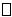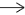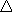{} {theme} theory: interpretations 2

## HOME-PAGE           SITE-MAP          EMAILFor the time being, this (very recent) page only contains material for further use.

A substitution reaction (also known as single displacement reaction or single substitution reaction) is a chemical reaction during which one functional group in a chemical compound is replaced by another functional group. Substitution reactions are of prime importance in organic chemistry. Substitution reactions in organic chemistry are classified either as electrophilic or nucleophilic depending upon the reagent involved, whether a reactive intermediate involved in the reaction is a carbocation, a carbanion or a free radical, and whether the substrate is aliphatic or aromatic. Detailed understanding of a reaction type helps to predict the product outcome in a reaction. It also is helpful for optimizing a reaction with regard to variables such as temperature and choice of solvent.

A good example of a substitution reaction is halogenation. When chlorine gas (Cl2) is irradiated, some of the molecules are split into two chlorine radicals (Cl•) whose free electrons are strongly nucleophilic. One of them breaks a C–H covalent bond in CH4 and grabs the hydrogen atom to form the electrically neutral HCl. The other radical reforms a covalent bond with the CH3• to form CH3Cl (methyl chloride).Benzene derivatives have from one to six substituents attached to the central benzene core. Examples of benzene compounds with just one substituent are phenol, which carries a hydroxyl group, and toluene with a methyl group. When there is more than one substituent present on the ring, their spatial relationship becomes important for which the arene substitution patterns orthometa, and para are devised.

An addition reaction, in organic chemistry, is in its simplest terms an organic reaction where two or more molecules combine to form a larger one (the adduct).

Addition reactions are limited to chemical compounds that have multiple bonds, such as molecules with carbon–carbon double bonds (alkenes), or with triple bonds (alkynes), and compounds that have rings, which are also considered points of unsaturation. Molecules containing carbon—hetero double bonds like carbonyl (C=O) groups, or imine (C=N) groups, can undergo addition, as they too have double-bond character.

An addition reaction is the reverse of an elimination reaction. For instance, the hydration of an alkene to an alcohol is reversed by dehydration.

Hydration, the addition of water across the double bond of alkenes, yields alcohols. The reaction is catalyzed by phosphoric acid or sulfuric acid. This reaction is carried out on an industrial scale to produce synthetic ethanol.

CH2=CH2 + H2O → CH3–CH2OH

An elimination reaction is a type of organic reaction in which two substituents are removed from a molecule in either a one- or two-step mechanism. The one-step mechanism is known as the E2 reaction, and the two-step mechanism is known as the E1 reaction. The numbers refer not to the number of steps in the mechanism, but rather to the kinetics of the reaction:rearrangement reaction is a broad class of organic reactions where the carbon skeleton of a molecule is rearranged to give a structural isomer of the original molecule. Often a substituent moves from one atom to another atom in the same molecule, hence these reactions are usually intramolecular.

In terms of the electronic nature of the molecule, aromaticity describes a conjugated system often made of alternating single and double bonds in a ring. This configuration allows for the electrons in the molecule's pi system to be delocalized around the ring, increasing the molecule's stability. The molecule cannot be represented by one structure, but rather a resonance hybrid of different structures, such as with the two resonance structures of benzene. These molecules cannot be found in either one of these representations, with the longer single bonds in one location and the shorter double bond in another  Rather, the molecule exhibits bond lengths in between those of single and double bonds.Representations of benzene.

X-ray diffraction shows that all six carbon-carbon bonds in benzene are of the same length, at 140 picometres (pm). The C–C bond lengths are greater than a double bond (135 pm) but shorter than a single bond (147 pm). This intermediate distance is caused by electron delocalization: the electrons for C=C bonding are distributed equally between each of the six carbon atoms. Benzene has 6 hydrogen atoms, fewer than the corresponding parent alkane, hexane, which has 14. Benzene and cyclohexane have a similar structure, only the ring of delocalized electrons and the loss of one hydrogen per carbon distinguishes it from cyclohexane. The molecule is planar. The molecular orbital description involves the formation of three delocalized π orbitals spanning all six carbon atoms, while the valence bond description involves a superposition of resonance structures. It is likely that this stability contributes to the peculiar molecular and chemical properties known as aromaticity. To accurately reflect the nature of the bonding, benzene is often depicted with a circle inside a hexagonal arrangement of carbon atoms.

a directed graph or digraph is an ordered pair G = (V, A) where

• V is a set whose elements are called vertices, nodes, or points;
• A is a set of ordered pairs of vertices, called arcs, directed edges (sometimes simply edges with the corresponding set named E instead of A), arrows, or directed lines.

An undirected graph  is defined in terms of unordered pairs of vertices,  usually called edges, links or lines.

n mathematics, physics, and engineering, a vector space (also called a linear space) is a set of objects called vectors, which may be added together and multiplied ("scaled") by numbers called scalars. Scalars are often real numbers, but some vector spaces have scalar multiplication by complex numbers or, generally, by a scalar from any mathematic field. The operations of vector addition and scalar multiplication must satisfy certain requirements, called vector axioms (listed below in § Notation and definition). To specify whether the scalars in a particular vector space are real numbers or complex numbers, the terms real vector space and complex vector space are often used.

Certain sets of Euclidean vectors are common examples of a vector space. They represent physical quantities such as forces, where any two forces of the same type can be added to yield a third, and the multiplication of a force vector by a real multiplier is another force vector. In the same way (but in a more geometric sense), vectors representing displacements in the plane or three-dimensional space also form vector spaces. Vectors in vector spaces do not necessarily have to be arrow-like objects as they appear in the mentioned examples: vectors are regarded as abstract mathematical objects with particular properties, which in some cases can be visualized as arrows.

Vector spaces are the subject of linear algebra and are well characterized by their dimension, which, roughly speaking, specifies the number of independent directions in the space. Infinite-dimensional vector spaces arise naturally in mathematical analysis as function spaces, whose vectors are functions.

Vector spaces

P. 690

A vector space over a field (of scalars) F is a set V (of vectors) together with an operation + on V and a mapping, called scalar multiplication, from the cartesian product F x V to V ... such tha the following conditions are satisfield for all scalars a, b [elements of] F and all vectors v,w [elements of] V.

Graph Theory and its applications
Jonathan L Gross and Jay Yellen

THe benzene molecule ... has double bonds for some pairs of its atoms, so it is modeled by a non-simple graph. Since each carbon atom has valence 4, corresponding to four electrons in the outer shell, it is represented by a vertex of degree 4, and since each hydrogen atom has one electron in its only shell, it is represented by a vertex of degree 1.

The simultaneous equations

x = 2y - 4,  2x = 3y - 5

can be solved by substituting for x in the second the expression with which it is equating in the first, giving 4y - 8 = 3y - 5, so y = 3.

List of {themes}:

{completion}{contrast} ( )
{direction}{distance} D
{diversification}{modification}